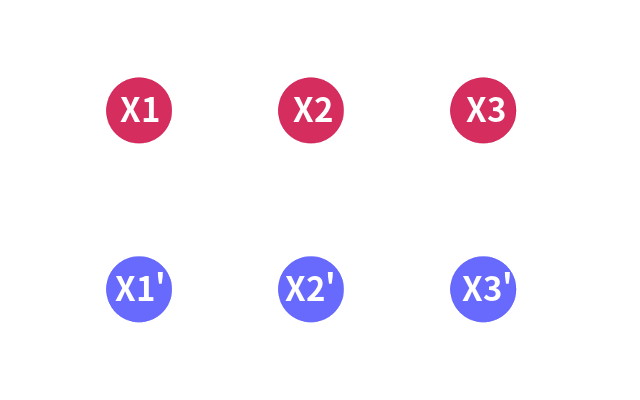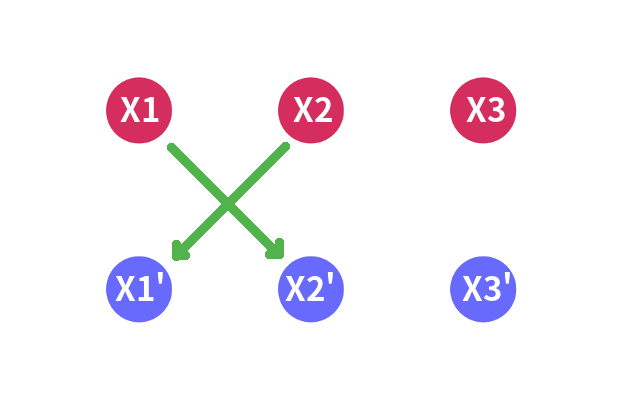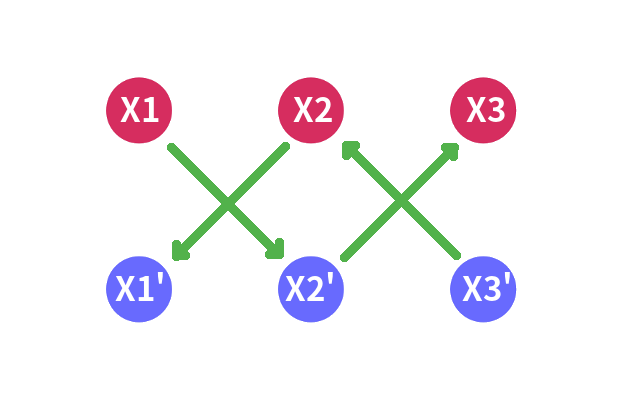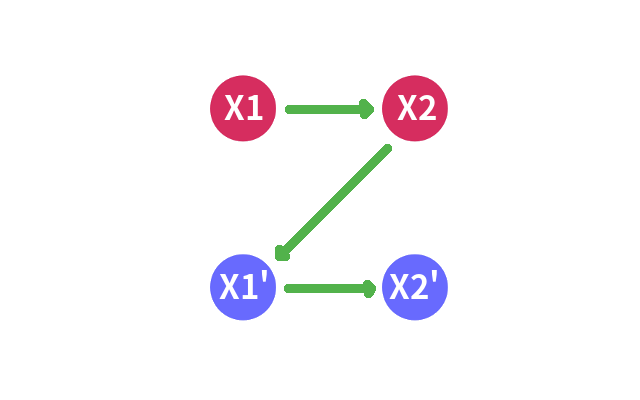# 什么是 2-SAT？

SAT 是适定性（Satisfiability）问题的简称。一般形式为 k – 适定性问题，简称 k-SAT。而当 $k > 2$ 时该问题为 NP 完全的。所以我们只研究 $k = 2$ 的情况。

# 如何解决# 算法# 例题

## 1. Panda’s Trick POJ – 3207

$n \leq 1000, m \leq 500$

$X _ i -> X _ j’$
$X _ i’ -> X _ j$
$X _ j – > X _ i’$
$X _ j’ -> X _ i$

#include <cstdio>
#include <cctype>
#include <cstring>
#include <stack>
#include <algorithm>

#define NS (2005)
#define MS (500000)

using namespace std;

template<typename _Tp> inline void IN(_Tp& dig)
{
char c; bool flag = 0; dig = 0;
while (c = getchar(), !isdigit(c)) if (c == '-') flag = 1;
while (isdigit(c)) dig = dig * 10 + c - '0', c = getchar();
if (flag) dig = -dig;
}

int n, m, X[NS], Y[NS], col[NS], cnt;

struct graph
{
graph() { init(); }
void push(int a, int b)
{
}
} g;

void dfs(int a)
{
col[a] = cnt;
for (int i = g.head[a]; ~i; i = g.nxt[i])
if (!col[g[i]]) dfs(g[i]);
}

int main(int argc, char const* argv[])
{
while (~scanf("%d%d", &n, &m))
{
for (int i = 1; i <= m; i += 1)
{
IN(X[i]), IN(Y[i]);
if (X[i] > Y[i]) swap(X[i], Y[i]);
}
for (int i = 1; i <= m; i += 1)
for (int j = i + 1; j <= m; j += 1)
{
if (X[i] == X[j] || Y[i] == Y[j])
{
puts("the evil panda is lying again");
goto end;
}
int a = i, b = j;
if (X[a] > X[b]) swap(a, b);
if (X[b] < Y[a] && Y[a] < Y[b])
{
g.push(a << 1, b << 1 | 1), g.push(b << 1 | 1, a << 1);
g.push(b << 1, a << 1 | 1), g.push(a << 1 | 1, b << 1);
}
}
for (int i = 1; i <= m; i += 1)
{
if (!col[i << 1]) cnt++, dfs(i << 1);
if (!col[i << 1 | 1]) cnt++, dfs(i << 1 | 1);
}
for (int i = 1; i <= m; i += 1)
if (col[i << 1] == col[i << 1 | 1])
{
puts("the evil panda is lying again");
goto end;
}
puts("panda is telling the truth...");
end : g.init(), memset(col, 0, sizeof(col)), cnt = 0;
}
return 0;
}


## 2. Wedding POJ – 3648

（编号从 $0$开始，结婚的新人是第 $0$对夫妻）

$n \leq 30$

$X _ 1 -> X _ 2$
$X _ 2′ -> X _ 1’$

#include <cstdio>
#include <cctype>
#include <cstring>
#include <stack>
#include <algorithm>

#define NS (65)
#define MS (3605)

using namespace std;

int n, m, id[NS], low[NS], dfn, scc[NS], cnt;

stack<int> st;

bool ins[NS];

struct graph
{
graph() { init(); }
void push(int a, int b)
{
}
} g;

void tarjan(int a)
{
id[a] = low[a] = ++dfn, st.push(a), ins[a] = 1;
for (int i = g.head[a]; ~i; i = g.nxt[i])
if (!id[g[i]]) tarjan(g[i]), low[a] = min(low[a], low[g[i]]);
else if (ins[g[i]]) low[a] = min(low[a], id[g[i]]);
if (id[a] == low[a])
{
scc[a] = ++cnt;
while (st.top() != a)
scc[st.top()] = cnt, ins[st.top()] = 0, st.pop();
ins[a] = 0, st.pop();
}
}

int main(int argc, char const* argv[])
{
while (scanf("%d%d", &n, &m), n || m)
{
for (int i = 1, a, b; i <= m; i += 1)
{
char ac, bc;
scanf("%d%c%d%c", &a, &ac, &b, &bc);
a++, b++, a <<= 1, b <<= 1;
if (ac == 'w') a |= 1;
if (bc == 'w') b |= 1;
g.push(a, b ^ 1), g.push(b, a ^ 1);
}
g.push(3, 2);
for (int i = 1; i <= n; i += 1)
{
if (!id[i << 1]) tarjan(i << 1);
if (!id[i << 1 | 1]) tarjan(i << 1 | 1);
}
for (int i = 1; i <= n; i += 1)
if (scc[i << 1] == scc[i << 1 | 1])
{ puts("bad luck"); goto end; }
for (int i = 2; i <= n; i += 1)
if (scc[i << 1] < scc[i << 1 | 1]) printf("%dw ", i - 1);
else printf("%dh ", i - 1);
putchar(10);
end : g.init(), memset(id, 0, sizeof(id)), dfn = cnt = 0;
}
return 0;
}


#### Remmina

No puzzle that couldn't be solved.